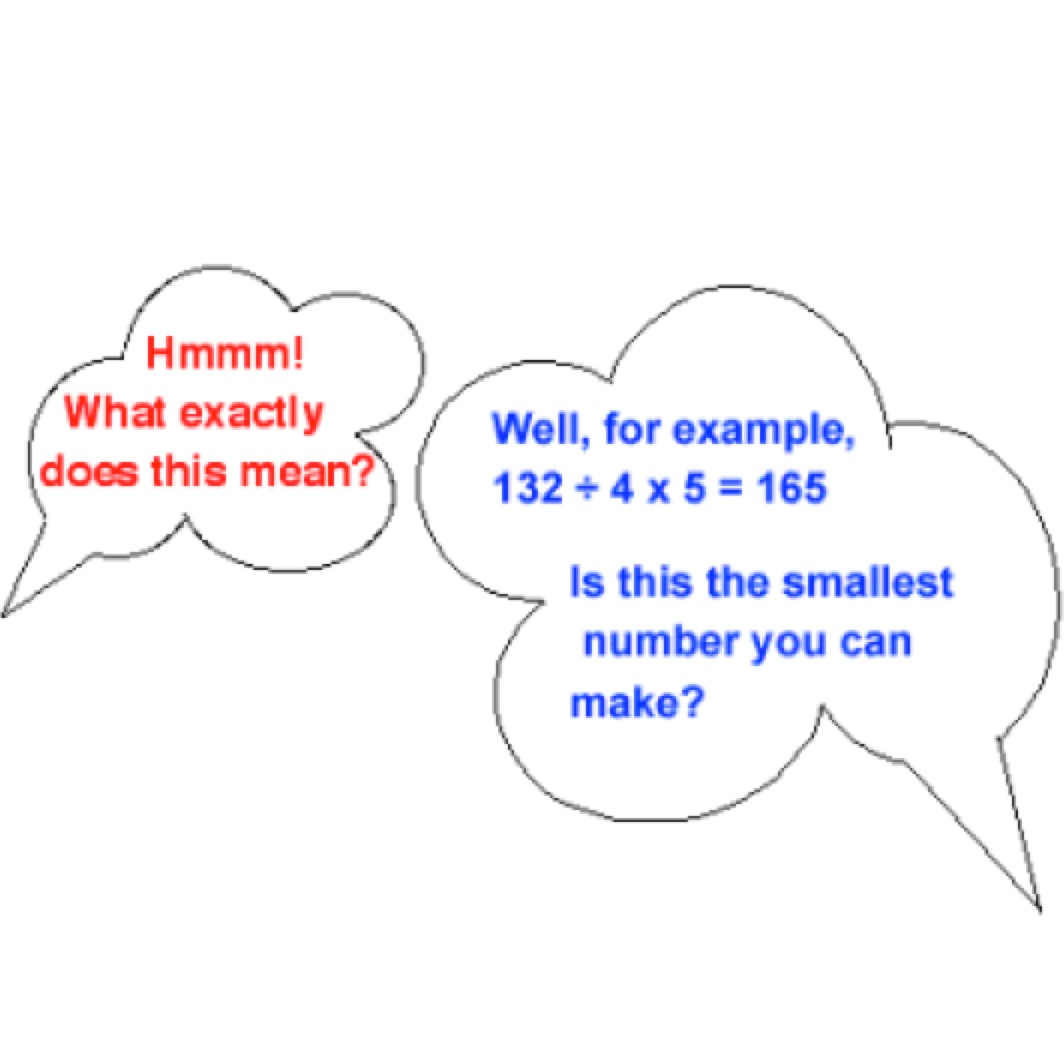Using the numbers 1, 2, 3, 4 and 5 once and only once and the symbols × and ÷  once and only once, what is the smallest whole number you can make?

Now suppose you have to use the symbol + once and the symbol ÷ twice what is the smallest fraction that you can find?

For example $\frac{1}{4}+\frac{2}{35}= \frac{43}{140}.$

Can you find a smaller fraction?

1.Manxesi Nxesi says: